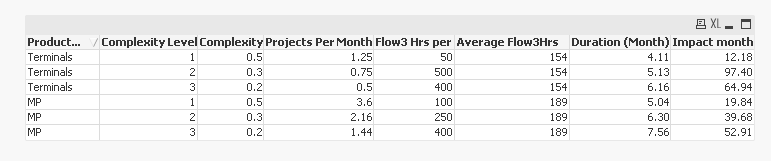# App Development

Discussion board where members can learn more about Qlik Sense App Development and Usage.

Announcements
April 22, 2PM EST: Learn about GeoOperations in Qlik Sense SaaS READ MORE
cancel
Showing results for
Search instead for
Did you mean:Creator

## Sum of Expression values

Hello Guys , i want to add below expression and create new column as Summation. Kindly let me know how to do ?

("AverageHrs"/30)*0.8)

("AverageHrs"/30)

(("AverageHrs"/30)*1.2))

I want to add values of all three expression and assign it new field as Summation

I tried like this but its not working

Sum((("Average Flow3Hrs"/30)*0.8)and ("Average Flow3Hrs"/30) and(("Average Flow3Hrs"/30)*1.2))

as summation,

Thanks,

ravi

19 RepliesMVP

Average Flow3Hrs comes as a field? Oh so then left join is not needed. Can you explain how you are getting 50, 500, 400 for the same field (Flow3 Hrs per) in a single row? A single row can have only one of the three values, right? Either 50 or 500 or 400... what is the logic for having different numbers for them?Creator
Author

Hey sunny ,

Please find the file attached .MVP

Okay this make a lot more sense, now how do you know if you need to multiply by 0.8, 1 or 1.2? Is this based on Complexity level?Creator
Author

yes yes thats assumptions and its fixed values.

I have attached another  excel file which has one more set of product line .

So how do i sum all Duration month and Impact month for both product line .

At the end i need only two values

Duration month and Impact month to plot a line chart

Using For loop ?Creator
Author

Sorry here is attachmentMVP

Try this script

Table:

LOAD *,

[Average Flow3Hrs] * Pick([Complexity Level], 0.8, 1, 1.2)/30 as [Duration (Month)],

[Flow3 Hrs per]/([Average Flow3Hrs] * Pick([Complexity Level], 0.8, 1, 1.2)/30) as [Impact month];

LOAD * INLINE [

Product Line, Complexity Level, Complexity, Projects Per Month, Flow3 Hrs per, Average Flow3Hrs

Terminals, 1, 0.5, 1.25, 50, 154

Terminals, 2, 0.3, 0.75, 500, 154

Terminals, 3, 0.2, 0.5, 400, 154

MP, 1, 0.5, 3.6, 100, 189

MP, 2, 0.3, 2.16, 250, 189

MP, 3, 0.2, 1.44, 400, 189

];

And then you can sum the Duration and Impact month on the front end, unless you need them in the script?Creator
Author

Thanks sunny will try itCreator
Author

(IF(("Complexity Level"='1'),("Flow3 Hrs per")/((("Average Flow3Hrs"/30)*0.8))))+

(IF(("Complexity Level"='2'),("Flow3 Hrs per")/ (("Average Flow3Hrs"/30))))+

(IF(("Complexity Level"='3'),("Flow3 Hrs per")/(("Average Flow3Hrs"/30)*1.2))) as "Total1",

this individual expression works in the script , but if i have to add all three expressions  it does not work .

is there any syntax ? specificMVP

You don't want to see the individual rows? Only see Aggregated data? Product and Final number will be the only two fields which go into your dashboard?Creator
Author

thanks sunny yes you are right ! It worked !With the reference to the above table which I have already attached  in spreadsheet .

I need to read each and every record of this table and fetch (Duration Month) and (Impact Month Value )

I have written a code kindly check the script and please do see comments .

[Project1]:
LOAD
"Product Line",
"Complexity Level",
Complexity,
"Projects Per Month",
"Flow3 Hrs per",
"Average Flow3Hrs",
"Duration (Month)",
"Impact month"

FROM [lib://APtiv documnets/Complexity_Mix_2.xlsx]
(ooxml, embedded labels, header is 1 lines, table is Model);

let vDATA_COUNT = NoOfRows('Project1');
Let vProductlines=Peek('Product Line',Project1,\$(n));
let vcomplexity=Peek('Complexity Level',Project1,\$(n));
Let vDurationMonthFlow3=Peek("Duration (Month)",,Project1,\$(n));
let vImpactmonthFlow3=Peek('Impact month',Project1,\$(n));

For n= 1 to \$(vDATA_COUNT)-1    // Should  read all the rows of the table  . Is it correct ?

For i=1 to 36   // Need Total months as 36

For x=1 to \$(vDurationMonthFlow3)   // Should read through all record values of ("Duration (Month)"

if \$(i) =1 then

test:
load
\$(i) as Month,
\$(x) as Month2,
\$(vFlow3Impact_Month) as value   // Should read all record values of 'Impact month'
AutoGenerate 1;

ELSE
test:
load
\$(i) as Month,
\$(x)+(\$(i)-1) as Month2,
\$(vFlow3Impact_Month) as value   // Should read all record values of 'Impact month'

AutoGenerate 1;
ENDIF

NEXT x;

Next i ;

Next n;

This code is perfect to my requirements . Its just That it needs some correction specially For Loop

Finally I need only Value and Month2 to draw my Line curve .

Thanks,

RaviTags